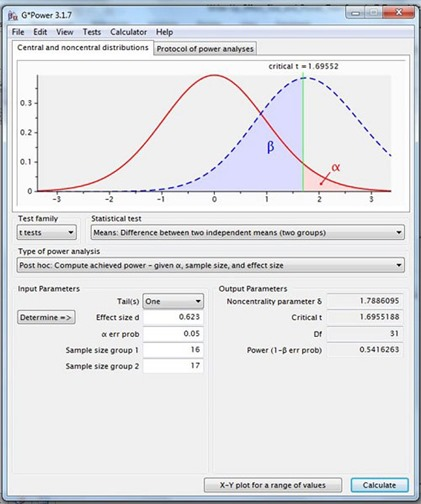## Friday, May 30, 2014

### 2-Sample Pooled t-Test Power With G*Power Utility

This is one of the following eight articles on 2-Independent-Sample Pooled t-Tests in Excel

2-Independent-Sample Pooled t-Test in 4 Steps in Excel 2010 and Excel 2013

Excel Variance Tests: Levene’s, Brown-Forsythe, and F Test For 2-Sample Pooled t-Test in Excel 2010 and Excel 2013

Excel Normality Tests Kolmogorov-Smirnov, Anderson-Darling, and Shapiro Wilk Tests For Two-Sample Pooled t-Test

Two-Independent-Sample Pooled t-Test - All Excel Calculations

2-Sample Pooled t-Test – Effect Size in Excel 2010 and Excel 2013

2-Sample Pooled t-Test Power With G*Power Utility

Mann-Whitney U Test in 12 Steps in Excel as 2-Sample Pooled t-Test Nonparametric Alternative in Excel 2010 and Excel 2013

2- Sample Pooled t-Test = Single-Factor ANOVA With 2 Sample Groups

# Power of 2-Sample Pooled t-Test With Free Utility G*Power

The Power of a two-independent-sample, pooled t-Test is a measure of the test’s ability to detect a difference given the following parameters:

Alpha (α)

Effect Size (d)

Sample Sizes (n1 and n2)

Number of Tails

Power is defined by the following formula:

Power = 1 – β

Β equals the probability of a Type 2 Error. A Type 2 Error can be described as a False Negative. A false Negative represents a test not detecting a difference when a difference does exist.

1 – β = Power = the probability of a test detecting a difference when one exists.

Power is therefore a measure of the sensitivity of a statistical test. It is common to target a Power of 0.8 for statistical tests. A Power of 0.8 indicates that a test has an 80 percent probability of detecting a difference.

The four variables that are required in order to determine the Power for a one-sample t-Test are Alpha (α), Effect Size (d), Sample Sizes (n1 and n2), and the Number of Tails. Typically alpha, Effect Size, and the Number of Tails are held constant while sample sizes are varied (usually increased) to achieve the desired Power for the statistical test.

Manual calculation of a test’s Power given Alpha, Effect Size, Sample Size, and the Number of Tails are quite tedious. Fortunately there are a number of free utilities online that will readily calculate a test’s statistical Power. A widely-used online Power calculation utility called G*Power is available for download from the Institute of Experimental Psychology at the University of Dusseldorf at this link:

http://www.psycho.uni-duesseldorf.de/abteilungen/aap/gpower3/

Screen shots will show how use this utility to calculate the Power for this example and also to provide a graph of Sample Size vs. Achieved Power for this example as follows:

As mentioned, the four variables that are required in order to determine Power for a one-sample t-Test are Alpha (α), Effect Size (d), Sample Size (n), and the Number of Tails.

Bring up G*Power’s initial screen and input the following information:

Test family: t-Tests

Statistical test: Means: Difference between two independent means (two groups)

Type of power analysis: Post hoc – Compute achieved power –given α, sample size, and effect size

Number of Tails = 1

Effect Size (d) = 0.623

Alpha (α) = 0.05

Sample Sizes (n1 = 16 and n2 = 17)

The completed dialogue screen appears as follows:(Click On Image To See a Larger Version)

Clicking Calculate would produce the following output:(Click On Image To See a Larger Version)

The Power achieved for this test is 0.5416. This means that the current one-tailed test has a 54.16 percent chance of detecting a difference that has an effect size of 0.623 if α = 0.05, n1 = 16, and n2 = 17.

It is often desirable to plot a graph of sample size versus achieve Power for the given Effect Size and alpha. This can be done by clicking the button X-Y plot for a range of values and then clicking Draw Plot on the next screen that comes up. This will produce the following output:(Click On Image To See a Larger Version)

This would indicate that a Power of 80 percent would be achieved for this test if the total sample size were equal to approximately n1 + n2 = 65.

Excel Master Series Blog Directory

Statistical Topics and Articles In Each Topic

• Histograms in Excel
• Bar Chart in Excel
• Combinations & Permutations in Excel
• Normal Distribution in Excel
• t-Distribution in Excel
• Binomial Distribution in Excel
• z-Tests in Excel
• t-Tests in Excel
• Hypothesis Tests of Proportion in Excel
• Chi-Square Independence Tests in Excel
• Chi-Square Goodness-Of-Fit Tests in Excel
• F Tests in Excel
• Correlation in Excel
• Pearson Correlation in Excel
• Spearman Correlation in Excel
• Confidence Intervals in Excel
• Simple Linear Regression in Excel
• Multiple Linear Regression in Excel
• Logistic Regression in Excel
• Single-Factor ANOVA in Excel
• Two-Factor ANOVA With Replication in Excel
• Two-Factor ANOVA Without Replication in Excel
• Randomized Block Design ANOVA in Excel
• Repeated-Measures ANOVA in Excel
• ANCOVA in Excel
• Normality Testing in Excel
• Nonparametric Testing in Excel
• Post Hoc Testing in Excel
• Creating Interactive Graphs of Statistical Distributions in Excel
• Solving Problems With Other Distributions in Excel
• Optimization With Excel Solver
• Chi-Square Population Variance Test in Excel
• Analyzing Data With Pivot Tables and Pivot Charts
• SEO Functions in Excel
• Time Series Analysis in Excel
• VLOOKUP
• Simplifying Useful Excel Functions